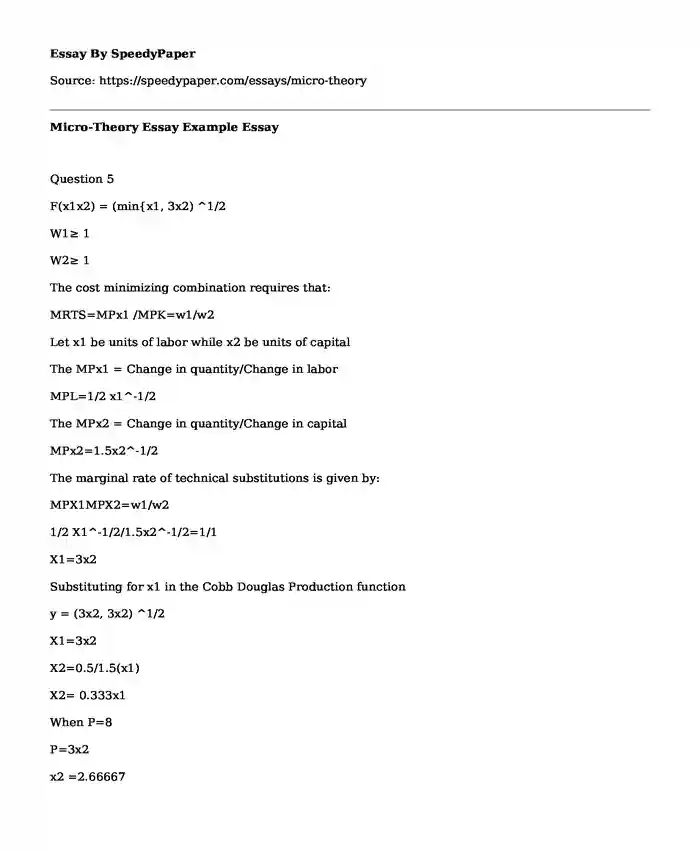# Micro-Theory Essay Example

Published: 2017-11-28Type of paper: Essay Categories: Economics Finance Pages: 2 Wordcount: 523 words
143 views

Question 5

Is your time best spent reading someone else’s essay? Get a 100% original essay FROM A CERTIFIED WRITER!

F(x1x2) = (min{x1, 3x2) ^1/2

W1≥ 1

W2≥ 1

The cost minimizing combination requires that:

MRTS=MPx1 /MPK=w1/w2

Let x1 be units of labor while x2 be units of capital

The MPx1 = Change in quantity/Change in labor

MPL=1/2 x1^-1/2

The MPx2 = Change in quantity/Change in capital

MPx2=1.5x2^-1/2

The marginal rate of technical substitutions is given by:

MPX1MPX2=w1/w2

1/2 X1^-1/2/1.5x2^-1/2=1/1

X1=3x2

Substituting for x1 in the Cobb Douglas Production function

y = (3x2, 3x2) ^1/2

X1=3x2

X2=0.5/1.5(x1)

X2= 0.333x1

When P=8

P=3x2

x2 =2.66667

X1=1.666

X2=0.333x1

X2= (0.333*1.666)

X2=0.5444

Q = (1.666, 3{0.5444}) ^1/2

Q= (2.7216)1/2

Q*=1.65 Units

Question 6

Question 6a

The long run formula will be :

Q(x1, x2)=min(x1,x2)^1/2

In the short run, the labor which is greater than 16 has no effect on the level of out put.

The short run production function is given by:

Y=

Y= 4 Therefore, L>4 or L≤ 4

The formula for the marginal product of labor

MPL= (4L)^-1/2 :L>4 or L≤ 4

Question 6b

When w=1 and price =\$ 4

The labor demand which is optimal=PMPL=w

It thus means 4*(1/2(L)^1/2=1

2/(L)^1/2=1

(L^1/2)^2=(2)^2

L=4

Question 6c

When w=1 and price =\$ 10

The labor demand which is optimal=PMPL=w

It thus means 4*(1/2(L)^1/2=1

5/(L)^1/2=1

(L^1/2)^2= (5)^2

L=25 Units

Question 6d

The equilibrium demand for labor PMPL=w

And in this situation w=p/2(L) ^1/2

Therefore, the equation can be written as:

L=min {(p/2w) ^2, 4}

Question 7

Question 7a

Total cost function=c=y^2+10 for y>0 and c=0

MR=MC

MC=First derivative of cost function

MC=MR=2y

The average cost= TC/Q

= (y^2+10)/y

= y + (10/y)

Question 7b

The minimum average cost

Av=(y^2+10)/y

First order derivatives

AV’=y+(10/y)

1+10/y

The minimum of MC

MC’=2y

But at Minimums MC=AV

1+10/y=2y

Y+10=2y2

2y2-y-10=0

y(2y-1)=10

y=10

0r

2y-1=10 Units

Y= 4.5 units

The quantity at which the quantity is minimized in the long run

The first derivative equated to 0

MC=MR=2y=0

Y=0/2= ∞

Hence the minimum is absolute minimum quantity.

Question 7c

MC=2y=MR

The variable cost= y2

Average variable cost= y2/y

=y which is minimized at y=0

Marginal function is given as

First derivative

oVC/dy=2y

P=2y which is the supply curve

At the quantity of 4.5

P= (2*4.5)

P = 9 Units

At the quantity of 10

P=2*(10)

P=20 Units

Therefore, the minimum price in which they can produce a positive quantity is 9 Units

Question 8

Question 8a

Given that s1(p) = p, s2(p) = 2p, s3(p) = 3p.

Then the market supply function is given as

S(P)= (S1+S2+S3)P

=S(p)=( p+2p+3p)

S(p)=6p

Question 8b

Given that s1(p) = 2p, s2(p) = p – 1

Then the market supply function is given as:

S(P)= (S1+S2)P

S(P)= (p – 1)+2p

S(P)=3P-1

Question 8c

200 firms each having a supply function of s1 (p) = 2p – 8 and other 100 firms having a supply function of s2 (p) = p – 3

The first 200 firms supply function

S1(p)=200(2p-8)

S(p)=400p-1600 …..1

The 2nd 200 firms supply function

The supply function = s2(p) = p – 3

(s(p) =100( p – 3)

(s(p)=100p-300………2

Total supply for the market is the summation of equation 1 and 2

Market’s s(p)= {400p-1600} +{100p-300}

s(p)= 500p-1900

s(p)=5p-19